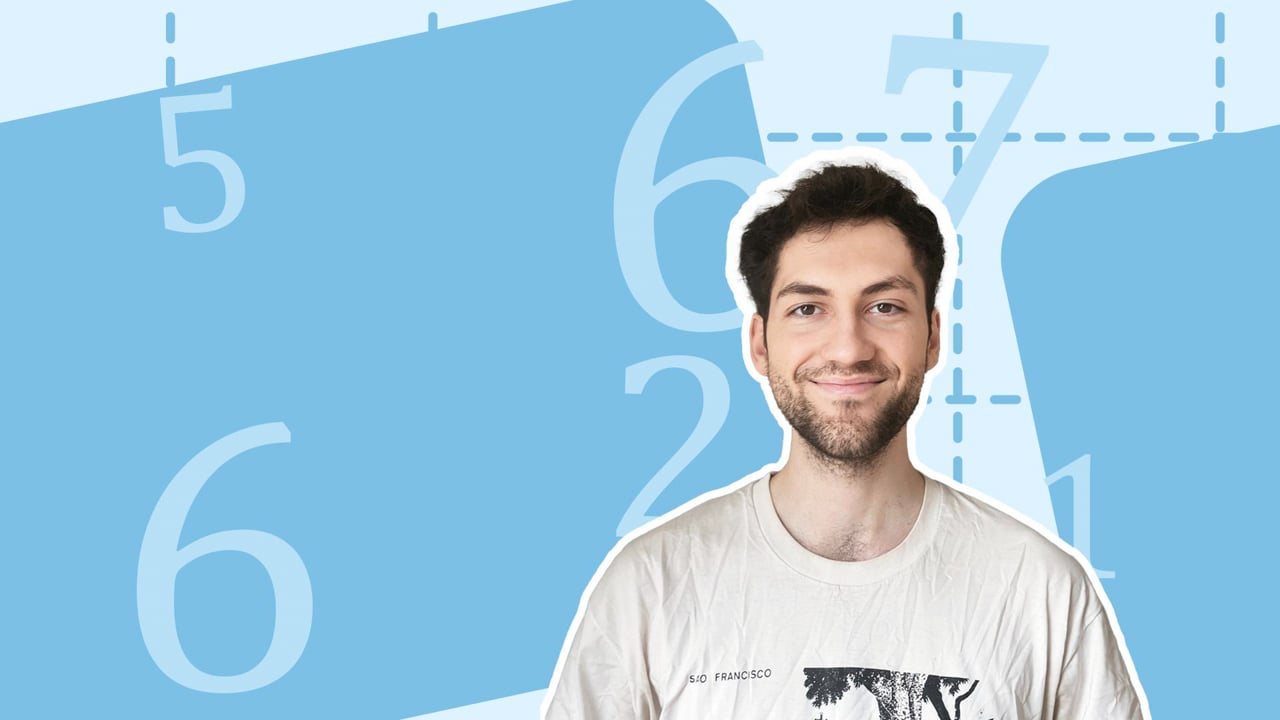Chapter overviewMaths

Number and place value

Multiplication and division

Fractions

Measurement

Geometry - properties of shapes

Geometry - position and direction

Statistics

Maths

# Equivalent fractions with tenths and hundredths0%

Summary

# Equivalent fractions with tenths and hundredths

## In a nutshell

The is no limit to the way a fraction can be expressed. Different looking fractions can represent the same value even if the numerators and denominators involved are multiples of tens or hundreds apart.

## Vocabulary reminder

The word equivalent means equal in value. So if two fractions are equivalent, they represent the same value.

##### Example 1

The following fractions are equivalent:

$\dfrac3{10}$ and $\dfrac{60}{200}$​​

## Showing equivalence

### Using common denominators

One way to check whether two fractions are equivalent is to make the denominators the same before comparing numerators. ​

##### Example 2

Are the following fractions equivalent?

$\dfrac4{10}$ and $\dfrac{90}{300}$​​

To make both denominators the same, pick a common denominator. Here you can use $300$:

$\dfrac4{10}=\dfrac{4\times30}{10\times30}=\dfrac{120}{300}$​​

Now compare numerators.

As $90$ and $120$ are not equal, $\dfrac4{10}$ and $\dfrac{90}{300}$ are not equivalent fractions.

### Simplifying fractions

Alternatively, simplifying fully will also tell you if two fractions are equivalent.

##### Example 3

Consider again the fractions:

$\dfrac3{10}$ and $\dfrac{60}{200}$​​

Their equivalence can be shown by fully simplifying each fraction. The first fraction is already fully simplified since there are no factors (other than $1$) in common between the numerator and the denominator. The second fraction can be simplified. This can be done in steps: first divide the numerator and denominator by $2$ and then by $10$:

$\dfrac{60}{200}=\dfrac{\cancel{60}^{\space\space30}}{\cancel{200}^{\space\space100}}=\dfrac{\cancel{30}^{\space\space3}}{\cancel{100}^{\space\space10}}=\dfrac3{10}$​​

As $\dfrac{60}{200}=\dfrac3{10}$, they are equivalent fractions.

## Want to find out more? Check out these other lessons!

Equivalent fractions

FAQs

• Question: How do you tell if two fractions are equivalent?

Answer: One way to check whether two fractions are equivalent is to make the denominators the same before comparing numerators. Alternatively, simplifying fully will also tell you if two fractions are equivalent.

• Question: What does equivalent mean?

Answer: In a mathematical context, the word equivalent means equal in value.

Theory

Exercises